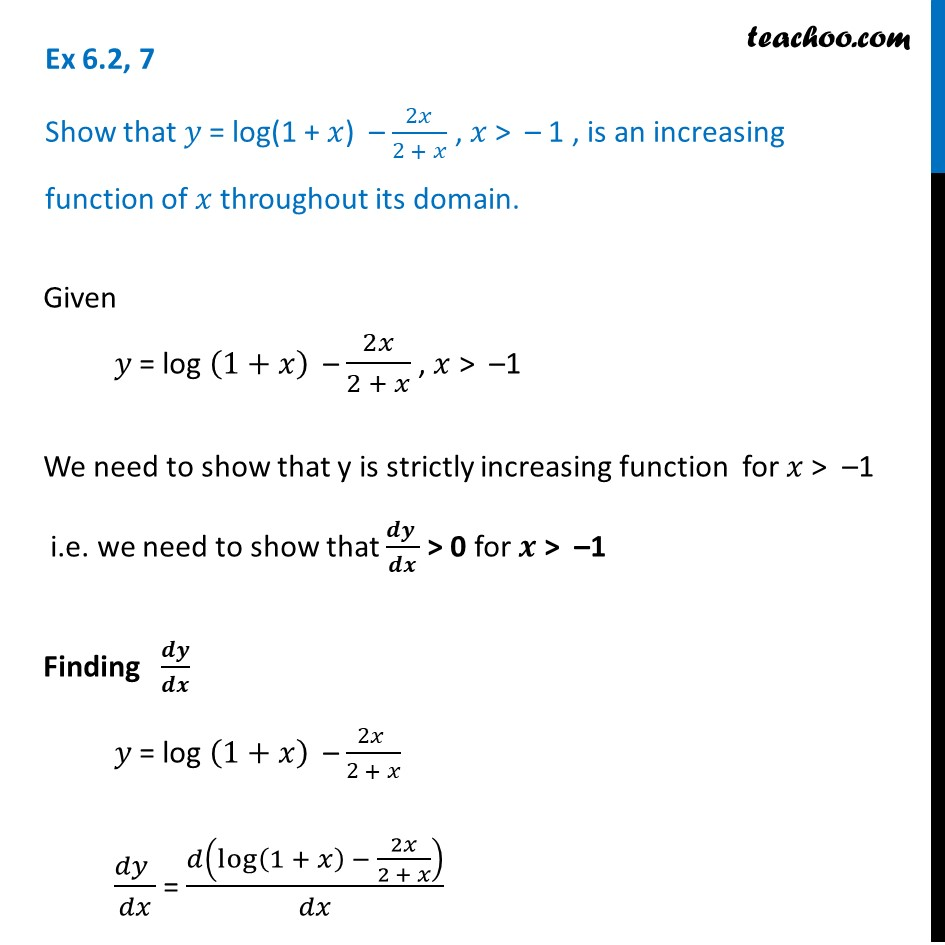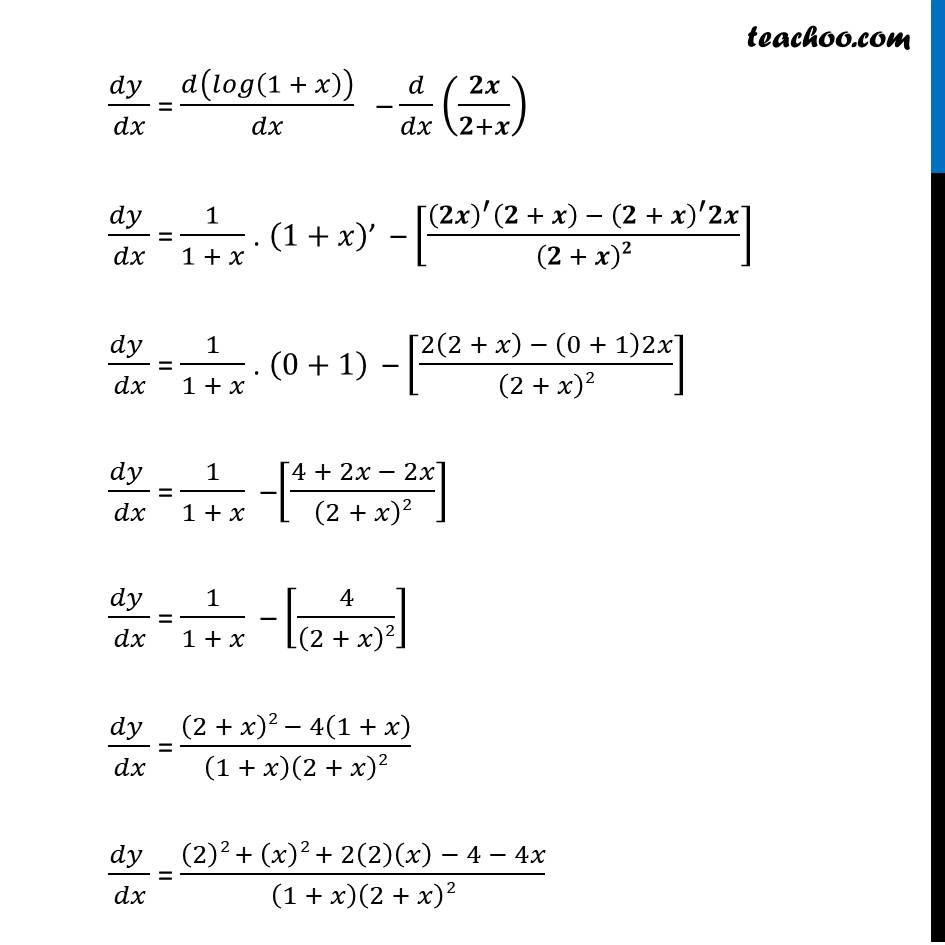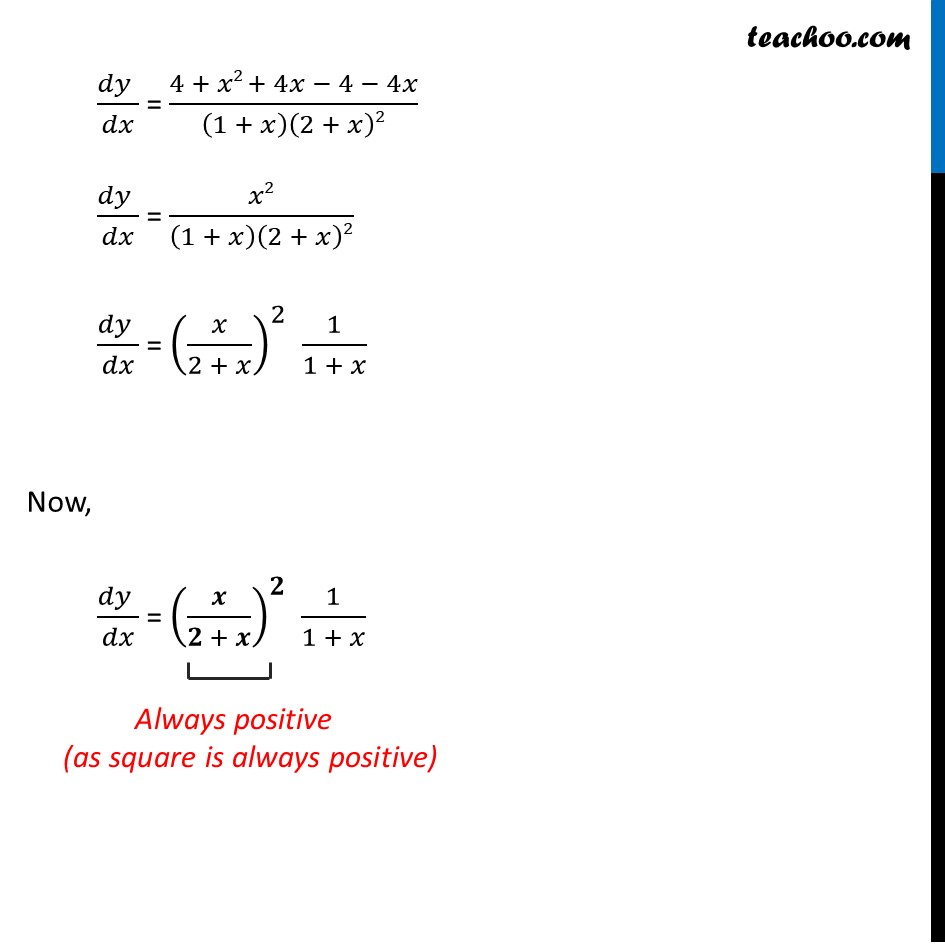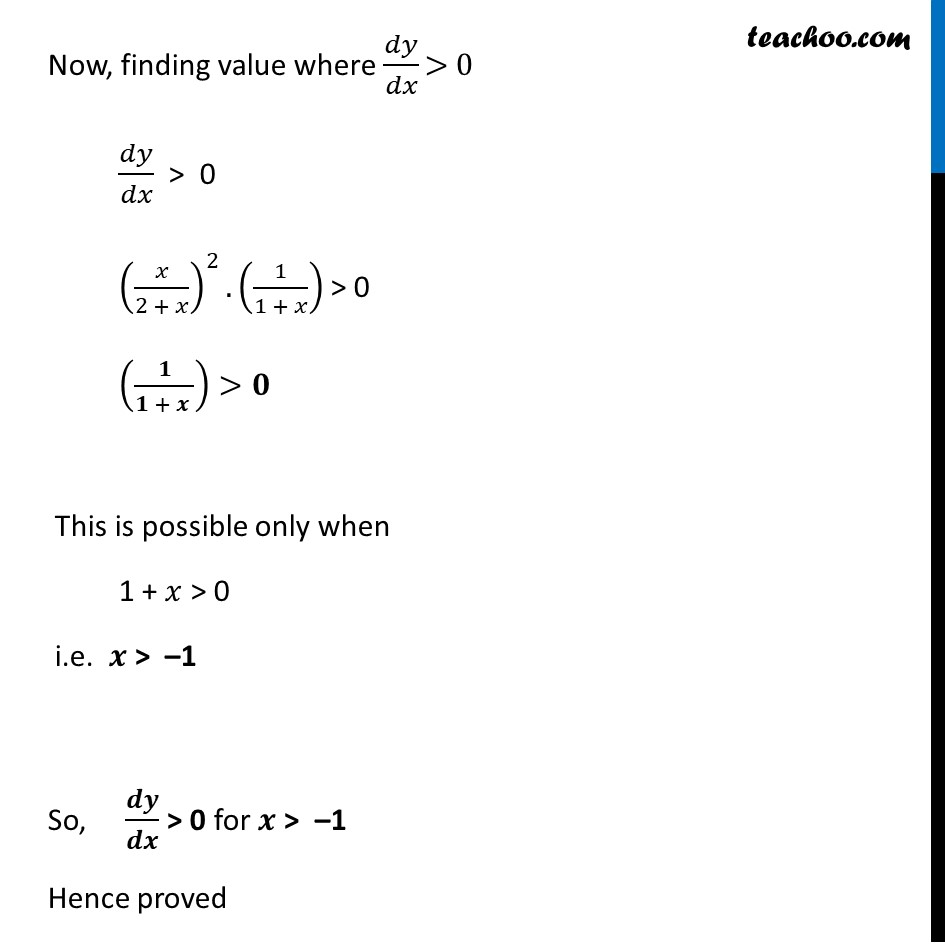Ex 6.2

Chapter 6 Class 12 Application of Derivatives
Serial order wiseLearn in your speed, with individual attention - Teachoo Maths 1-on-1 Class

### Transcript

Ex 6.2, 7 Show that 𝑦 = log(1 + 𝑥) – 2𝑥/(2 + 𝑥) , 𝑥 > – 1 , is an increasing function of 𝑥 throughout its domain.Given 𝑦 = log (1+𝑥) – 2𝑥/(2 + 𝑥) , 𝑥 > –1 We need to show that y is strictly increasing function for 𝑥 > –1 i.e. we need to show that (𝒅𝒚 )/𝒅𝒙 > 0 for 𝒙 > –1 Finding 𝒅𝒚/𝒅𝒙 𝑦 = log (1+𝑥) – (2𝑥 )/(2 + 𝑥) (𝑑𝑦 )/𝑑𝑥 = 𝑑(log⁡〖(1 + 𝑥) − 2𝑥/(2 + 𝑥)〗 )/𝑑𝑥 (𝑑𝑦 )/𝑑𝑥 = 𝑑(𝑙𝑜𝑔(1 + 𝑥))/𝑑𝑥 – 𝑑/𝑑𝑥 (𝟐𝒙/(𝟐+𝒙)) (𝑑𝑦 )/𝑑𝑥 = 1/(1 + 𝑥) . (1+𝑥)’ – [((𝟐𝒙)^′ (𝟐 + 𝒙) −〖 (𝟐 + 𝒙)〗^′ 𝟐𝒙)/(𝟐 + 𝒙)𝟐] (𝑑𝑦 )/𝑑𝑥 = 1/(1 + 𝑥) . (0+1) – [(2(2 + 𝑥) − (0 + 1)2𝑥)/(2 + 𝑥)2] (𝑑𝑦 )/𝑑𝑥 = 1/(1 + 𝑥) –[(4 + 2𝑥 − 2𝑥)/(2 + 𝑥)2] (𝑑𝑦 )/𝑑𝑥 = 1/(1 + 𝑥) – [4/(2 + 𝑥)2] (𝑑𝑦 )/𝑑𝑥 = ((2 + 𝑥)2 − 4(1 + 𝑥))/(1 + 𝑥)(2 + 𝑥)2 (𝑑𝑦 )/𝑑𝑥 = ((2)2 + (𝑥)2 + 2(2)(𝑥) − 4 − 4𝑥)/(1 + 𝑥)(2 + 𝑥)2 (𝑑𝑦 )/𝑑𝑥 = (4 + 𝑥2 + 4𝑥 − 4 − 4𝑥)/(1 + 𝑥)(2 + 𝑥)2 (𝑑𝑦 )/𝑑𝑥 = 𝑥2/(1 + 𝑥)(2 + 𝑥)2 (𝑑𝑦 )/𝑑𝑥 = (𝑥/(2 + 𝑥))^2 1/(1 + 𝑥) Now, (𝑑𝑦 )/𝑑𝑥 = (𝒙/(𝟐 + 𝒙))^𝟐 1/(1 + 𝑥) Now, finding value where 𝑑𝑦/𝑑𝑥 > 0 𝑑𝑦/𝑑𝑥 > 0 (𝑥/(2 + 𝑥))^2.(1/(1 + 𝑥)) > 0 (𝟏/(𝟏 + 𝒙 ))>𝟎 This is possible only when 1 + 𝑥 > 0 i.e. 𝒙 > –1 So, 𝒅𝒚/𝒅𝒙 > 0 for 𝒙 > –1 Hence proved